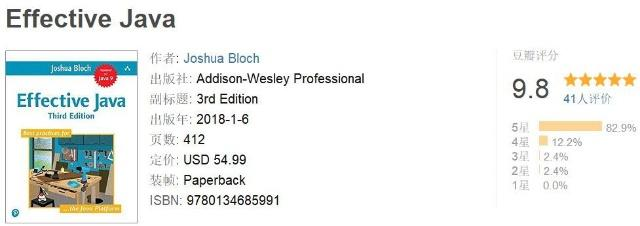# 带你快速看完9.8分神作《Effective Java》—— Lambda 和 Stream篇（小王工作里用的很多）🍊 Java学习：Java从入门到精通总结## 42 Lambda优先于匿名类

``````Collections.sort(words, new Comparator<String>() {
public int compare(String s1, String s2) {
return Integer.compare(s1.length(), s2.length());
}
});``````

``Collections.sort(words, (s1, s2) -> Integer.compare(s1.length(), s2.length()));``

Lambda的类型是Comparator <String>，其参数s1，s2的类型是String，返回值类型int全都没有出现在代码里

``Collections.sort(words, comparingInt(String::length));``

``words.sort(comparingInt(String::length));``

Java中增加了Lambda之后，使得之前不能使用函数对象的地方现在也能用了。例如，以34条里的Operation枚举类型为例：

``````public enum Operation {
PLUS("+") {
public double apply(double x, double y) {
return x + y;
}
},
MINUS("-") {
public double apply(double x, double y) {
return x - y;
}
},
TIMES("*") {
public double apply(double x, double y) {
return x * y;
}
},
DIVIDE("/") {
public double apply(double x, double y) {
return x / y;
}
};

private final String symbol;

Operation(String symbol) {
this.symbol = symbol;
}

@Override
public String toString() {
return symbol;
}

public abstract double apply(double x, double y);
}``````

``````public enum Operation {
PLUS("+", (x, y) -> x + y),
MINUS("-", (x, y) -> x - y),
TIMES("*", (x, y) -> x * y),
DIVIDE("/", (x, y) -> x / y);

private final String symbol;
private final DoubleBinaryOperator op;

Operation(String symbol, DoubleBinaryOperator op) {
this.symbol = symbol;
this.op = op;
}

@Override
public String toString() {
return symbol;
}

public double apply(double x, double y) {
return op.applyAsDouble(x, y);
}
}``````

Lambda表达式一行是最好的，三行是极限！不能再多了

2. 如果一个接口有多个抽象方法，创建实例要用匿名类

3. Lambda无法获得对自身的引用 在Lambda中，关键字this指向外围实例，这通常是我们想要的

## 43 方法引用优先于Lambda

Java提供了一种生成函数对象的方法，比lambda还要简洁：方法引用（method references），就是常说的::运算符

``map.merge(key, 1, (count, incr) -> count + incr);``

Integer 类（和所有其他包装数字基本类型）提供了一个静态方法sum，只传入这个方法的引用也行：

``map.merge(key, 1, Integer::sum);``

- 方法引用

``service.execute(GoshThisClassNameIsHumongous::action);``

- Lambda

``service.execute(() -> action());``

``x -> x``

## 44 优先使用标准的函数式接口

``````protected boolean removeEldestEntry(Map.Entry<K,V> eldest) {
return size() > 100;
}``````

``````@FunctionalInterface
interface EldestEntryRemovalFunction<K,V>{
boolean remove(Map<K,V> map, Map.Entry<K,V> eldest);
}``````

EldestEntryRemovalFunction 接口使用@FunctionalInterface注解进行标注的。这个注解类型本质上与@Override一样，有三个目的：

1. 告诉读者这个接口是针对Lambda设计的
2. 这个接口不会进行编译，除非他只有一个抽象方法
3. 避免后续维护人员不小心给该接口添加抽象方法

• 这六个基础接口各自还有3种变体（int、long、double），例如predicate的变体IntPredicate
• Function 接口还有9种变体，LongToIntFunction、DoubleToObjFunction等
• 这三种基础函数接口还有带两个参数的版本，BiPredicate <T，U>、BiFunction <T，U，R>、BiConsumer <T，U>
• 还有BiFunction变体用于返回三个相关的基本类型：ToIntBiFunction<T，U>，ToLongBiFunction<T，U> 和ToDoubleBiFunction <T，U>
• Consumer接口也有带两个参数的变体版本，带一个对象和一个基本类型：ObjDoubleConsumer <T>，ObjIntConsumer <T>和 ObjLongConsumer <T>
• 还有一个 BooleanSupplier 接口，它是 Supplier 的一个变体，返回boolean

1. 每当在API中使用时，其名称提供了良好的文档信息
2. Comparator接口对于如何构成一个有效的实例，有着严格的条件限制
<li>这个接口配置了大量好用的default方法，可以对Comparator进行转换和合并</li>

1. 通用，并且将受益于描述性的名称
2. 具有与其关联的严格的契约
3. 将受益于定制的缺省方法

## 45 谨慎使用Stream

Stream表示有限或无限的数据元素序列，Stream pipeline，表示对这些元素的多级计算。Stream中的元素可以来自集合、数组、文件、正则表达式模式匹配器、伪随机数生成器和其他Stream。数据可以是对象引用或基本类型（int、long、double）。

• Stream pipeline是lazy的：直到调用终止操作时才会开始计算

• Stream API是fluent的：所有包含pipeline的调用可以链接成一个表达式

``````public class Anagrams {
public static void main(String[] args) throws IOException {
File dictionary = new File(args);
int minGroupSize = Integer.parseInt(args);
Map<String, Set<String>> groups = new HashMap<>();
try (Scanner s = new Scanner(dictionary)) {
while (s.hasNext()) {
String word = s.next();
groups.computeIfAbsent(alphabetize(word),

}
}

for (Set<String> group : groups.values())
if (group.size() >= minGroupSize)
System.out.println(group.size() + ": " + group);
}

private static String alphabetize(String s) {
char[] a = s.toCharArray();
Arrays.sort(a);
return new String(a);
}
}``````

``hashmap.computeIfAbsent(K key, Function remappingFunction)``

• key - 键
• remappingFunction - 重新映射函数，用于重新计算value

``````public class Anagrams {
public static void main(String[] args) throws IOException {
Path dictionary = Paths.get(args);
int minGroupSize = Integer.parseInt(args);
try (Stream<String> words = Files.lines(dictionary)) {
words.collect(
groupingBy(word -> word.chars().sorted()
.collect(StringBuilder::new,
(sb, c) -> sb.append((char) c),
StringBuilder::append).toString()))
.values().stream()
.filter(group -> group.size() >= minGroupSize)
.map(group -> group.size() + ": " + group)
.forEach(System.out::println);
}
}
}``````

``````public class Anagrams {

public static void main(String[] args) throws IOException {
Path dictionary = Paths.get(args);
int minGroupSize = Integer.parseInt(args);

try (Stream<String> words = Files.lines(dictionary)) {
words.collect(groupingBy(word -> alphabetize(word)))
.values().stream()
.filter(group -> group.size() >= minGroupSize)
.forEach(g -> System.out.println(g.size() + ": " + g));
}
}

// alphabetize method is the same as in original version
private static String alphabetize(String s) {
char[] a = s.toCharArray();
Arrays.sort(a);
return new String(a);
}
}``````

``values().stream()``

• 在没有显式类型的情况下，认真命名Lambda参数
• 使用辅助方法（上面的alphabetize），因为pipeline缺少显式类型信息和命名临时变量

``"Hello world!".chars().forEach(System.out::print);``

``"Hello world!".chars().forEach(x -> System.out.print((char) x));``

• 统一转换元素序列
• 过滤元素序列
• 使用单个操作组合元素序列(例如添加、连接或计算最小值)
• 将元素序列累积到一个集合中，可能通过一些公共属性将它们分组
• 在元素序列中搜索满足某些条件的元素

``````private static List<Card> newDeck() {
List<Card> result = new ArrayList<>();

for (Suit suit : Suit.values())
for (Rank rank : Rank.values())

return result;
}``````

``````private static List<Card> newDeck() {
return Stream.of(Suit.values())
.flatMap(suit ->
Stream.of(Rank.values())
.map(rank -> new Card(suit, rank)))
.collect(toList());
}``````

newDeck的两个版本中到底哪一个更好？这就是仁者见仁智者见智的问题了，取决于你的个人喜好 : )

## 46 优先选择Stream中无副作用的函数

Stream最重要的是把将计算结构构造成一系列变型，其中每个阶段的结果尽可能接近前一阶段结果的纯函数（pure function）。纯函数的结果仅取决于其输入的函数：它不依赖于任何可变状态，也不更新任何状态。为了实现这一点，Stream操作的任何中间操作和终结操作都应该是没有副作用的。

``````Map<String, Long> freq = new HashMap<>();
try (Stream<String> words = new Scanner(file).tokens()) {
words.forEach(word -> {
freq.merge(word.toLowerCase(), 1L, Long::sum);
});
}``````

``````Map<String, Long> freq;
try (Stream<String> words = new Scanner(file).tokens()) {
freq = words
.collect(groupingBy(String::toLowerCase, counting()));
}``````

``````List<String> topTen = freq.keySet().stream()
.sorted(comparing(freq::get).reversed())
.limit(10)
.collect(toList());``````

1. toMap(keyMapper、valueMapper)

``````private static final Map<String, Operation> stringToEnum =
Stream.of(values()).collect(toMap(Object::toString, e -> e));``````

``Map<Artist, Album> topHits = albums.collect(toMap(Album::artist, a->a, maxBy(comparing(Album::sales))));``2. groupingBy

``words.collect(groupingBy(word -> alphabetize(word)))``

``Map<String, Long> freq = words.collect(groupingBy(String::toLowerCase, counting()));``

3. groupingByConcurrent

4. joining

## 47 Stream要优先用Collection作为返回类型

• 如果可以返回集合，就返回集合
• 如果集合中已经有元素，或者元素数量不多，就返回一个标准集合，比如 ArrayList
• 否则，就需要自定义集合，如下文将提到的幂集
• 如果不能返回集合，则返回Stream或Iterable

``for (ProcessHandle ph : (Iterable<ProcessHandle>)ProcessHandle.allProcesses()::iterator)``

``````public static <E> Iterable<E> iterableOf(Stream<E> stream) {
return stream::iterator;
}``````

``````for (ProcessHandle p : iterableOf(ProcessHandle.allProcesses())) {
// Process the process
}``````

``````public static <E> Stream<E> streamOf(Iterable<E> iterable) {
return StreamSupport.stream(iterable.spliterator(), false);
}``````

Collection接口是Iterable的子类型，有一个stream方法，因此提供了迭代和stream访问。所以<font color="#3333ff">Collection或适当的子类型通常是公共序列返回方法的最佳返回类型</font>。

<!-- -->{}，{a}，{b}，{c}，{a，b}，{a，c}，{b，c}，{a，b, c}}

``````public class PowerSet {
public static final <E> Collection<Set<E>> of(Set<E> s) {
List<E> src = new ArrayList<>(s);

if (src.size() > 30)
throw new IllegalArgumentException("Set too big " + s);

return new AbstractList<Set<E>>() {
@Override
public int size() {
return 1 << src.size(); // 2 to the power srcSize
}

@Override
public boolean contains(Object o) {
return o instanceof Set && src.containsAll((Set)o);
}

@Override
public Set<E> get(int index) {
Set<E> result = new HashSet<>();
for (int i = 0; index != 0; i++, index >>= 1)
if ((index & 1) == 1)
return result;
}
};
}
}``````

## 48 谨慎使用Stream并行

``````public static void main(String[] args) {
primes().map(p -> TWO.pow(p.intValueExact()).subtract(ONE))
.filter(mersenne -> mersenne.isProbablePrime(50))
.limit(20)
.forEach(System.out::println);
}

static Stream<BigInteger> primes() {
return Stream.iterate(TWO, BigInteger::nextProbablePrime);
}``````

`TWO.pow(p.intValueExact()).subtract(ONE))`表示2 ^ p - 1
`mersenne.isProbablePrime(50)`表示mersenne是否可能是素数，传入的数字表示可能性阈值

2. 在顺序处理时提供了较好的引用局部性（localityof reference）

``````static long pi(long n) {
return LongStream.rangeClosed(2, n)
.parallel()
.mapToObj(BigInteger::valueOf)
.filter(i -> i.isProbablePrime(50))
.count();
}``````

-- 展开阅读全文 --

« 上一篇 07-24